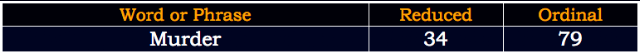## Thursday, January 26, 2017

### 59 | Bessie Coleman, the first recognized African-American female pilot's Google Doogle, January 26, 2017Atlanta, Texas?  That's on the 33rd Parallel just like Atlanta, Georgia.  It is very interesting the Atlanta Falcons play next Sunday in the Super Bowl in Texas...

Also, 'Bessie' died young, and I think I know why.  Keep in mind the over / under for the Super Bowl is at 59 points...

http://freetofindtruth.blogspot.com/2017/01/17-59-59-point-over-under-for-super.html

59 is the 17th prime number.

Bessie = 2+5+1+1+9+5 = 23/41
Coleman = 3+6+3+5+4+1+5 = 27
Bessie Coleman = 50/68 (America = 50)

Bessie = 2+5+19+19+9+5 = 59 (Negro) (Slave) (Blues) (Kill)
Coleman = 3+15+12+5+13+1+14 = 63
Bessie Coleman = 122

1/26/1892 = 1+26+18+92 = 137 (137, the 33rd prime)
1/26/1892 = 1+26+(1+8+9+2) = 47 (Foundation) (Framework)
1/26/1892 = 1+2+6+1+8+9+2 = 29
1/26/92 = 1+26+92 = 119 (All Seeing Eye) (Star of David) (Master Plan)
1/26 = 1+26 = 27

4/30/1926 = 4+30+19+26 = 79 (Murder)
4/30/1926 = 4+30+(1+9+2+6) = 52 (Prophecy) (Government)
4/30/1926 = 4+3+0+1+9+2+6 = 25
4/30/26 = 4+30+26 = 60
4/30 = 4+30 = 34 (Murder)She died 3-months and 4-days after her 34th birthday.

Ninety-Four = 57/147
World Series = 57/147
*Super Bowl 51 is connected to 112th World Series; Houston = 112

Today is the 91-year anniversary of her death.  Recall, when you sum 1 through 13, it totals 91.

2017 will mark the 114-year anniversary of the discovery of flight.

1.I think the GG is even making a 69 for the fly-by.

Permission to Buzz the Tower??

2.I can't come off the 97 / 25 thing. Falcons = 25
I sure hope they do win.

3.They will. Not expecting a close game.

4.#33 for the Patriots, the man with the '52' name gematria, is born 131-days before the Super Bowl. He worries me for you Falcons fans.

5.Not a fan. Just a local. I am really hoping for a nuclear attack from Aliens led by a Serpent Christ at Halftime, but we all have a dream.

6.And I think I said this before, but Falcons are Female. An Oriontholgist would call a Male Falcon a Tercel.

Terence Mathis wore 88 and played for the 96 Falcons.

7.Anniversary of her death is 84 days after the Superbowl and was 9 months 6 days before the Superbowl. Jesuit numbers and Falcon numbers.